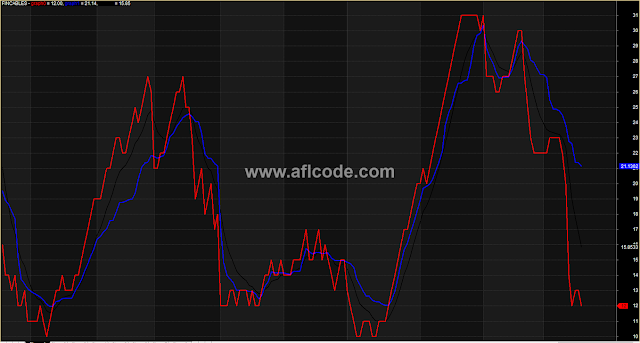### Trend Reversal ConfirmationTrend Reversal Confirmation

```//www.aflcode.com

EnableScript("VBScript");
value1=((H+L)/2) - Ref(((H+L)/2),-6);
value2= Ref(value1,-3);
value3=0.75*(value1-Ref(value1,-6)) + 0.25*(Ref(value1,-2)-Ref(value1,-4));

<%
value1 = AFL("Value1")
value2 = AFL("Value2")
value3 = AFL("Value3")
Close = AFL("Close")
inphase = Close

Call Setup()

function Setup()
for i = 1 to Ubound(Close)
inphase(i) =  0.33 * value2(i) + (0.67 * inphase(i-1) )
next
End function

AFL.Var("inphase") = inphase
%>
p1  =  atan(
)
*     360 /3.1416;
phase = IIf(inphase<0 AND quad>0, 180-p1,
dp  =  IIf(Ref(phase,-1)<90 AND phase>270, 360+Ref(phase,-1)-phase,Ref(phase,-1)-phase);
dp2 = IIf(dp < 1, 1,
IIf(dp > 60, 60, dp));

Graph1 = dp2;

HilbertCyclePeriod1a = dp2;
value = dp2;

HCycleCount1a=
IIf(Sum(value,6)>=360 AND Sum(value,5)<360 ,6,0) +
IIf(Sum(value,7)>=360 AND Sum(value,6)<360 ,7,0) +
IIf(Sum(value,8)>=360 AND Sum(value,7)<360 ,8,0) +
IIf(Sum(value,9)>=360 AND Sum(value,8)<360 ,9,0) +
IIf(Sum(value,10)>=360 AND Sum(value,9)<360 ,10,0) +
IIf(Sum(value,11)>=360 AND Sum(value,10)<360 ,11,0) +
IIf(Sum(value,12)>=360 AND Sum(value,11)<360 ,12,0) +
IIf(Sum(value,13)>=360 AND Sum(value,12)<360 ,13,0) +
IIf(Sum(value,14)>=360 AND Sum(value,13)<360 ,14,0) +
IIf(Sum(value,15)>=360 AND Sum(value,14)<360 ,15,0);

HCycleCount2a =
IIf(Sum(value,16)>=360 AND Sum(value,15)<360 ,16,0) +
IIf(Sum(value,17)>=360 AND Sum(value,16)<360 ,17,0) +
IIf(Sum(value,18)>=360 AND Sum(value,17)<360 ,18,0) +
IIf(Sum(value,19)>=360 AND Sum(value,18)<360 ,19,0) +
IIf(Sum(value,20)>=360 AND Sum(value,19)<360 ,20,0) +
IIf(Sum(value,21)>=360 AND Sum(value,20)<360 ,21,0) +
IIf(Sum(value,22)>=360 AND Sum(value,21)<360 ,22,0) +
IIf(Sum(value,23)>=360 AND Sum(value,22)<360 ,23,0) +
IIf(Sum(value,24)>=360 AND Sum(value,23)<360 ,24,0) +
IIf(Sum(value,25)>=360 AND Sum(value,24)<360 ,25,0);

HCyclecount3a =
IIf(Sum(value,26)>=360 AND Sum(value,25)<360 ,26,0) +
IIf(Sum(value,27)>=360 AND Sum(value,26)<360 ,27,0) +
IIf(Sum(value,28)>=360 AND Sum(value,27)<360 ,28,0) +
IIf(Sum(value,29)>=360 AND Sum(value,28)<360 ,29,0) +
IIf(Sum(value,30)>=360 AND Sum(value,29)<360 ,30,0) +
IIf(Sum(value,31)>=360 AND Sum(value,30)<360 ,31,0) +
IIf(Sum(value,32)>=360 AND Sum(value,31)<360 ,32,0) +
IIf(Sum(value,33)>=360 AND Sum(value,32)<360 ,33,0) +
IIf(Sum(value,34)>=360 AND Sum(value,33)<360 ,34,0) +
IIf(Sum(value,35)>=360 AND Sum(value,34)<360 ,35,0);

c1=  HCycleCount1a + HCycleCount2a + HCycleCount3a;
/*
graph0 = HCycleCount1a;
graph1 = HCyclecount2a;
Graph2 = HCyclecount3a;
Graph0Style=Graph1Style=Graph2Style=5;
*/
<%
c1 = AFL("c1")
c2 = c1
c3 = c1
for i = 1 to Ubound(c1)
if c1(i) = 0 then c2(i) = c2(i-1) else c2(i) = c1(i)
c3(i) = 0.25*c2(i) + 0.75*c3(i-1)
next

%>

GraphXSpace = 1;

/* playing a little here with moving averages, the Hilbert curve
using Amibroker seems a lot more jagged than the one
produced using Metastock.  I do not understand this
difference but using a moving average produces a curve
that is closer to Metastocks results than the one that I
produced as an interpetation.  */

fast = 2/(2+1);
slow = 2/(30+1);
dir=abs(Close-Ref(Close,-5));
vol=Sum(abs(Close-Ref(Close,-1)),5);
ER=dir/vol;
sc =( ER*(fast-slow)+slow)^2;

Graph0 = c1;
Graph1 = AMA( c1, sc );
Graph2 = EMA(c1,9);

Graph0Style=Graph1Style=Graph2Style=4;

/* Squelch  here is used as 15 instead of 20 on the site */
Squelch = 15;

var1 = Graph2;
Buy = IIf(var1 < Squelch , 0 , 1);
Sell = IIf( var1 < Squelch ,  1, 0 );

/* Exploration code */

/* filter is setup so that you can test any particular date and
see the followup performance.  Just make sure that that
date is at least 3 * first days in the past from the current
date, otherwise the future references will produce the
wrong information or no information useful */

first=1;
/* First is the number of days for each ROC interval for reviewing performance */

NumColumns = 7;
Column0 = C;
Column0Format = 1.2;
Column0Name = "Close";
Column1 = Ref(C, 1+first);
Column1Name = "Close+i ";
Column1Format = 1.2;
Column2 = Ref(C,1+first*2);
Column2Name = "Close+i*2   ";
Column2Format = 1.2;
Column3 =  Ref(C,1+first*3);
Column3Name = "Close+i*3 ";
Column3Format = 1.2;
Column4= Ref(C,first*1+1)  -  C;
Column4Name="ROC+i";
Column4Format = 1.2;
Column5=  Ref(C,first*2+1)  -  C;
Column5Name="ROC+2i";
Column5Format = 1.2;
Column6=  Ref(C,first*3+1)  -  C;
Column6Name="ROC+3i";
Column6Format = 1.2;

/*  End of Exploration Code. */
```

Previous
Next Post »